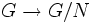# Coinflation functor on homology

Suppose$G$ is a group and$N$ is a normal subgroup of$G$. Suppose$A$ is an abelian group and$\varphi:G \to \operatorname{Aut}(A)$ is a homomorphism of groups, making$A$ into a$G$-module. Denote by$A_N$ the quotient of$A$ by the subgroup of elements of the form$\varphi(g)a - a$, with$g \in N$.
Then, the coinflation homomorphism$\operatorname{coinf}: H_*(G,A) \to H_*(G/N,A_N)$ is defined as the composite:$H_*(G,A) \to H_*(G,A/N) \stackrel{\operatorname{cor}}{\to} H_*(G/N,A_N)$
where the first map is the natural map obtained by viewing cohomology as a covariant functor in its second coordinate, applied to the surjection$A \to A_N$ of$G$-modules, and the second map is obtained by applying the corestriction functor on homology to the quotient map$G \to G/N$.